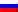# Cycle-canceling algorithm

Contenus

## Cycle-canceling algorithm

The cycle-canceling algorithm begins with maximum flow resolution. Then, iteratively, the algorithm seeks a cycle with a negative cost (negative cost if the edge is taken in the opposite direction to the flow) and increases the flow on this cycle. When there is no longer a negative cycle, the algorithm ends.

Example :

Let's build the gap graph and look for a negative cycle:

The 4-2-3-4 cycle has a cost of -3 + 1 + 1 = -1, we can saturate it with a flow of 2.

Similarly with the 4-2-1-3-4 cycle with a cost of -3 -2 +2 +1 = -2, we can saturate it with a flow of 1.

There is no longer a negative cycle, the algorithm ends.FRFRENRUES